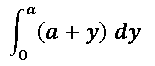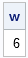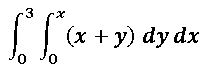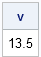The SAS/IML language provides the QUAD function for evaluating one-dimensional integrals. You can also use the QUAD function to compute a double integral as an iterated integral.

### A One-Dimensional Integration

Suppose you want to evaluate the following integral:To evaluate this integral in the SAS/IML language:

1. Define a function module that evaluates the integrand at an arbitrary point y.
2. Call the QUAD routine to numerically integrate the function over the domain of integration.

The module needs to be a function of a single variable (the integration variable). If the integral contains parameters, pass those in by using the GLOBAL clause to the START statement. For example, the following statements evaluate the integral for the parameter a=2:

```proc iml; start inner(y) global(a); return(a+y); finish;   /** evaluate integral for the parameter value, a=2 **/ a = 2; yinterval = 0 || a; call quad(w, "inner", yinterval); print w;```### Iterated Integrals

An iterated integral is a double integral in which you treat the variable in the outer integral as a constant while you evaluate the inner integral. If the domain of integration is not rectangular, the limits of integration for the inner integral are functions of the outer variable.

For example, consider the following iterated integral:Notice that the inner integral is exactly the integral that you computed in the previous section, except that the parameter is called x instead of a. You already know how to evaluate the inner integral, so to evaluate the double integral:

1. Define a function module that evaluates the integrand of the outer integral at an arbitrary point x. This module must internally evaluate the inner integral, which is a function of x.
2. Call the QUAD routine to numerically integrate the function over the domain of integration.

The following statements implement this approach:

```start outer(x) global(a); a = x; yinterval = 0 || a; /** evaluate inner integral for the parameter value, a=x **/ call quad(w, "inner", yinterval); return (w); finish;   xinterval= {0 3}; call quad(v, "outer", xinterval); /** outer integral **/ print v;```ShareDistinguished Researcher in Computational Statistics

Rick Wicklin, PhD, is a distinguished researcher in computational statistics at SAS and is a principal developer of SAS/IML software. His areas of expertise include computational statistics, simulation, statistical graphics, and modern methods in statistical data analysis. Rick is author of the books Statistical Programming with SAS/IML Software and Simulating Data with SAS.

1.I never realized that IML can do double integrals. I finally understand the QUAD syntax and why to use the GLOBAL statment. Thanks!

2.How can you do more than 2 integrals?

3.Rick Wicklin on

I assume you mean a triple integral or a d-dimensional integral. For higher-dimensional integrals, researchers usually use Monte Carlo integration. Monte-Carlo techniques don't converge very quickly (O(1/sqrt(N))) but that's typically the best you can do. When you have good knowledge of the function that you are integrating, you can use a variation known as "importance sampling," which can reduce the variance in your estimate.

4.Hello.....
Can I do triple integration in SAS 9.1? My 3 integration limits are all constants & two of them have infinity in the limits. I have used the QUAD function before in performing a single numerical integration. Could I use it in my triple integration?

Aya

•You can try. First remember that is one integral is a standard probability density (normal, gamma, weibull,...) then you should use the CDF function instead of QUAD. For the 2D normal probability density, use the PROBBNRM function. Some researchers suggest Monte Carlo approaches are better for triple integrals on an infiniate domain, so that would be a second possibility.

By the way, SAS has released SAS 9.4, so it might be time for an upgrade. A lot has changed in 10 years.

•Thank you for your continious help. My triple integration is as follows:
f(x)g(y)h(z)w(x,y,z) where x follows N(0,1), y follows chi distribution and z follows uniform(a,b) and the function w is computed using a certain module. Could you clarify more how could this be done using sas? It would be great if you give me an example.

Thanks
Aya

•This blog post provides you with an example. For your problem, each of the integrals is the same. For example, the x integral is Int(f(x)*w(x,y,z)) where y and z are CONSTANTS (=parameters). Figure out how to solve each 1D integral, then work on the iterated integral.

•Hello ......,

Here is the log file of my program, I tried it on a double integration but it gave me errors. Could you help me to figure out the problem

2 proc iml;

•You can submit programs and ask for help at the SAS/IML Support Community.

5.Multiple Integrals

1. Estimate sin (xy) dx dy where R is the square 0 x 1, 0 y 1 by using the Riemann sum corresponding to partition of R in four equal sub squares and with the function evaluated at their centers. Use three decimal places in the calculations. Ans: 0.242

2. Give the Riemann sum approximation of corresponding to the partition of R = {(x, y): 0 x 3, 0 y 3} into nine equal squares and with the integrand evaluated at their centers. Use two-decimal-place accuracy. Ans: 22.62

•You posted 12 homework problems, but there was no question in your post. If you wish to learn how to compute double integrals in SAS, read this article and the QUAD documentation. If you are stuck on a particular problem, post your attempt to the SAS/IML Support Community.

•in fact the questions exposed the above have most importance as is seen

6.these integral are very imp;ortant in spherical coordinates

7.Dear Dr Rick
I need to doubly integrate the bivariate normal distribution of normal (0,1) and another normal variate having nonzero positive mean and variance. The correlation is unknown and will have to be treated as a variable . So it’s a function of x, y and correlation. The integration ranges are finite for both x and y. The integral will this be a function of correlation . Can I use quad subroutine or by any way in sas iml to get this function? I need this function to be maximised using sas nlin to get a maximum likelihood estimate of correlation.

•For integration, you can use the PROBBNRM function, which evaluates the CDF of the bivariate normal distribution. See how to evaluate the bivariate CDF.
However, I don't understand why you need to perform any integration: the MLE estimate of the normal distribution is the sample mean and the sample covariance (without the Bessel adjustment) of the data. So if X is bivariate, mean(X) and (n-1)/n*cov(X) are the MLE estimates.

8.Dear Dr Rick
Thank you for your prompt response. I have to doubly integrate the bivariate normal density function of x and y with an unknown polyserial correlation, for eventually finding the maximum likelihood estimate of
the polyserial correlation for complex survey. The cdf can’t be used as it’s not a full -ve infinity to +ve infinity limit for the integration according to the R manual for wcorr package for polyserial correlation. The limits are determined based on summing survey weights wrt to different cut points. I want to develop the program for sas although it’s available in R wcorr package. Looking forward to your help.
Regards
Haider

•I am unable to provide personal help, but I will point out that the integral of f over the interval [a,b] equals the integral on (-infinity, b) minus the integral from (-infinity, a). I will also mention that polyserial correlation is available in SAS by using PROC CORR. Good luck.